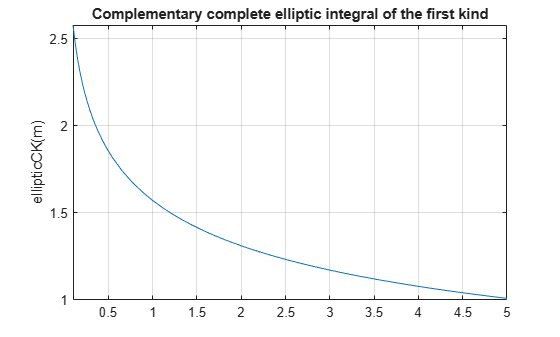# ellipticCK

Complementary complete elliptic integral of the first kind

## Syntax

``ellipticCK(m)``

## Description

example

````ellipticCK(m)` returns the complementary complete elliptic integral of the first kind.```

## Examples

### Find Complementary Complete Elliptic Integral of First Kind

Compute the complementary complete elliptic integrals of the first kind for these numbers. Because these numbers are not symbolic objects, you get floating-point results.

`s = [ellipticCK(1/2), ellipticCK(pi/4), ellipticCK(1), ellipticCK(inf)]`
```s = 1.8541 1.6671 1.5708 NaN```

Compute the complete elliptic integrals of the first kind for the same numbers converted to symbolic objects. For most symbolic (exact) numbers, `ellipticCK` returns unresolved symbolic calls.

```s = [ellipticCK(sym(1/2)), ellipticCK(sym(pi/4)),... ellipticCK(sym(1)), ellipticCK(sym(inf))]```
```s = [ ellipticCK(1/2), ellipticCK(pi/4), pi/2, ellipticCK(Inf)]```

Use `vpa` to approximate this result with floating-point numbers:

`vpa(s, 10)`
```ans = [ 1.854074677, 1.667061338, 1.570796327, NaN]```

### Differentiate Complementary Complete Elliptic Integral of First Kind

Differentiate these expressions involving the complementary complete elliptic integral of the first kind:

```syms m diff(ellipticCK(m)) diff(ellipticCK(m^2), m, 2)```
```ans = ellipticCE(m)/(2*m*(m - 1)) - ellipticCK(m)/(2*m - 2) ans = (2*(ellipticCE(m^2)/(2*m^2 - 2) -... ellipticCK(m^2)/(2*m^2 - 2)))/(m^2 - 1) -... (2*ellipticCE(m^2))/(m^2 - 1)^2 -... (2*ellipticCK(m^2))/(2*m^2 - 2) +... (8*m^2*ellipticCK(m^2))/(2*m^2 - 2)^2 +... (2*m*((2*m*ellipticCK(m^2))/(2*m^2 - 2) -... ellipticCE(m^2)/(m*(m^2 - 1))))/(2*m^2 - 2) -... ellipticCE(m^2)/(m^2*(m^2 - 1))```

Here, `ellipticCE` represents the complementary complete elliptic integral of the second kind.

### Find Elliptic Integral for Matrix Input

Call `ellipticCK` for this symbolic matrix. When the input argument is a matrix, `ellipticCK` computes the complementary complete elliptic integral of the first kind for each element.

`ellipticCK(sym([pi/6 pi/4; pi/3 pi/2]))`
```ans = [ ellipticCK(pi/6), ellipticCK(pi/4)] [ ellipticCK(pi/3), ellipticCK(pi/2)]```

### Plot Complementary Complete Elliptic Integral of First Kind

Plot complementary complete elliptic integral of first kind.

```syms m fplot(ellipticCK(m),[0.1 5]) title('Complementary complete elliptic integral of the first kind') ylabel('ellipticCK(m)') grid on hold off```## Input Arguments

collapse all

Input, specified as a number, vector, matrix, or array, or a symbolic number, variable, array, function, or expression.

collapse all

### Complementary Complete Elliptic Integral of the First Kind

The complementary complete elliptic integral of the first kind is defined as K'(m) = K(1–m), where K(m) is the complete elliptic integral of the first kind:

`$K\left(m\right)=F\left(\frac{\pi }{2}|m\right)=\underset{0}{\overset{\pi /2}{\int }}\frac{1}{\sqrt{1-m{\mathrm{sin}}^{2}\theta }}d\theta$`

Note that some definitions use the elliptical modulus k or the modular angle α instead of the parameter m. They are related as m = k2 = sin2α.

## Tips

• `ellipticK` returns floating-point results for numeric arguments that are not symbolic objects.

• For most symbolic (exact) numbers, `ellipticCK` returns unresolved symbolic calls. You can approximate such results with floating-point numbers using the `vpa` function.

• If `m` is a vector or a matrix, then `ellipticCK(m)` returns the complementary complete elliptic integral of the first kind, evaluated for each element of `m`.

 Milne-Thomson, L. M. “Elliptic Integrals.” Handbook of Mathematical Functions with Formulas, Graphs, and Mathematical Tables. (M. Abramowitz and I. A. Stegun, eds.). New York: Dover, 1972.